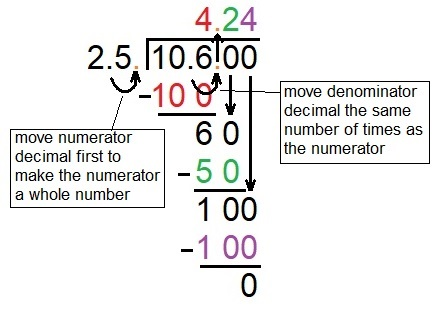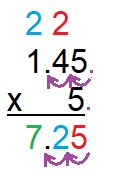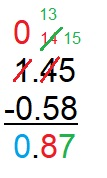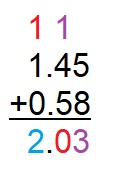# Mathematical Operations Flashcards

Mathematical Operations Flashcards
1/30 (missed) 0 0
Create Your Account To Continue Studying

As a member, you'll also get unlimited access to over 79,000 lessons in math, English, science, history, and more. Plus, get practice tests, quizzes, and personalized coaching to help you succeed.

Try it risk-free for 30 days. Cancel anytime
Divide 5/6 by 1/3 and simplify.

(5/6) ÷ (1/3) = (5/6) * (3/1) = 15/6 = 2 3/6 = 2 1/2

Got it
Divide 5/13 by 10 and simplify.

5/13 ÷ 10 = (5/13) * (1/10) = 5/130 = 1/26

Got it
Find the reciprocal of 4/17 and simplify.

1/(4/17) = 17/4 = 4 1/4

Got it
Reciprocal

The result of dividing the number one by a number

Example: the reciprocal of 5 is 1/5; the reciprocal of 3/5 is 1/(3/5) or 5/3

Got it
Divide 9 by 1/3.

9 ÷ 1/3 = 9 * 3/1 = 27

Got it
Solve the expression and express in simplest terms: 7/8 * 3

(7/8) * 3 = 21/8 = 2 5/8

Got it
Solve and simplify the expression: 5/6 - 1/6

5/6 - 1/6 = 4/6 = 2/3

Got it
Solve and simplify the expression: 2 4/5 + 9/10

2 4/5 = 14/5 = 28/10

28/10 + 9/10 = 37/10 = 3 7/10

Got it
Divide 10.6 by 2.5.Got it
Solve the expression: 1.45 * 5Got it
Solve the expression: 1.45 - 0.58Got it
Solve the expression: 1.45 + 0.58Got it
Solve the expression: (5 - 3)^3 * 4

Order of operations: PE(MD)(AS)

Parentheses: 5 - 3 = 2, expression is now 2^3 * 4

Exponent: 2^3 = 8, expression is now 8 * 4

Multiplication: 8 * 4 = 32

Got it
Order of Operations

The order of steps to solve mathematical expressions

PE(MD)(AS): Please Excuse My Dear Aunt Sally

Stands for parentheses, exponents, multiplication and division, addition and subtraction

Got it
Steps for Solving Mathematical Expressions

Simplify parentheses, simplify exponents, simplify multiplication and division from left to right, simplify addition and subtraction from left to right

Got it

or choose a specific lesson: See all lessons in this chapter
30 cards in set

## Flashcard Content Overview

When you see a complicated mathematical expression, the first thing to do is identify the operations present and determine the order in which they need to be solved. These flashcards will help you review that process. They will also help you review how to deal with tricky situations involving rational numbers, including decimals, improper fractions, and mixed numbers.

Front
Back
Steps for Solving Mathematical Expressions

Simplify parentheses, simplify exponents, simplify multiplication and division from left to right, simplify addition and subtraction from left to right

Order of Operations

The order of steps to solve mathematical expressions

PE(MD)(AS): Please Excuse My Dear Aunt Sally

Stands for parentheses, exponents, multiplication and division, addition and subtraction

Solve the expression: (5 - 3)^3 * 4

Order of operations: PE(MD)(AS)

Parentheses: 5 - 3 = 2, expression is now 2^3 * 4

Exponent: 2^3 = 8, expression is now 8 * 4

Multiplication: 8 * 4 = 32

Solve the expression: 1.45 + 0.58Solve the expression: 1.45 - 0.58Solve the expression: 1.45 * 5Divide 10.6 by 2.5.Solve and simplify the expression: 2 4/5 + 9/10

2 4/5 = 14/5 = 28/10

28/10 + 9/10 = 37/10 = 3 7/10

Solve and simplify the expression: 5/6 - 1/6

5/6 - 1/6 = 4/6 = 2/3

Solve the expression and express in simplest terms: 7/8 * 3

(7/8) * 3 = 21/8 = 2 5/8

Divide 9 by 1/3.

9 ÷ 1/3 = 9 * 3/1 = 27

Reciprocal

The result of dividing the number one by a number

Example: the reciprocal of 5 is 1/5; the reciprocal of 3/5 is 1/(3/5) or 5/3

Find the reciprocal of 4/17 and simplify.

1/(4/17) = 17/4 = 4 1/4

Divide 5/13 by 10 and simplify.

5/13 ÷ 10 = (5/13) * (1/10) = 5/130 = 1/26

Divide 5/6 by 1/3 and simplify.

(5/6) ÷ (1/3) = (5/6) * (3/1) = 15/6 = 2 3/6 = 2 1/2

Inverse Operations

An operation that cancels out another operation

Example inverse operation pairs: multiplication-division, addition-subtraction, exponent-root, logarithm-10^x

Find the inverse operation for the expression 5^3.

Inverse of 5^3 = cube root of 125

5^3 = 125; cube root of 125 = 5

Improper Fraction

A fraction in which the number in the numerator is greater than the number in the denominator

Determine which of the following are improper fractions: 11/5; 2/4; 4/3; 5/10

Improper fractions: numerator > denominator

11/5 and 4/3 are improper fractions

Convert to an improper fraction: 4 1/3

3 * 4 = 12 + 1 = 13

4 1/3 = 13/3

Convert to a mixed number: 18/7

18 ÷ 7 = 2 remainder 4

18/7 = 2 4/7

Mixed Number

A fraction that includes a whole number in addition to a numerator and a denominator

Solve the expression: (1/3) * (2/9)

1 * 2 = 2

3 * 9 = 27

(1/3) * (2/9) = 2/27

Solve the expression: 2 1/5 * 4

2 1/5 = 11/5

4 = 4/1

11 * 4 = 44

5 * 1 = 5

(11/5) * 4 = 44/5 = 8 4/5

Solve the expression: 6 1/3 * 5 1/2

6 1/3 = 19/3

5 1/2 = 11/2

(19/3) * (11/2) = 209/6 = 34 5/6

Divide 5/6 by 1/4.

(5/6) ÷ (1/4) = (5/6) * (4/1)

5 * 4 = 20

6 * 1 = 6

(5/6) ÷ (1/4) = 20/6 = 3 2/6 = 3 1/3

Divide 5 1/6 by 4 1/2.

5 1/6 = 31/6

4 1/2 = 9/2

(31/6) ÷ (9/2) = (31/6) * (2/9)

31 * 2 = 62

6 * 9 = 54

(5 1/6) ÷ (4 1/2) = 62/54 = 1 8/54 = 1 4/27

Find the number of books read in a year if a person reads 5 1/3 books in every month except October.

Number of months: 12/year - 1 = 11 months

Expression: 5 1/3 * 11

5 1/3 = 16/3

11 = 11/1

16 * 11 = 176

3 * 1 = 3

5 1/3 * 11 = 176/3 = 58 2/3 books

Find how many cups of flour are needed to make 5 cakes if each cake requires 1 3/4 cups of flour.

Expression: 1 3/4 * 5

1 3/4 = 7/4

5 = 5/1

7 * 5 = 35

4 * 1 = 4

1 3/4 * 5 = 35/4 = 8 3/4 cups of flour

Three people are sewing. Person A uses 3 1/4 yards of fabric. Person B uses 3 1/2 more yards of fabric than person A and 2 1/2 less yards than person C. Find the fabric amount used by B and C.

A: 3 1/4 yards = 13/4 yards

B = A + 3 1/2 yards = 13/4 + 7/2 = 13/4 + 14/4 = 27/4 = 6 3/4 yards

C = B + 2 1/2 yards = 27/4 + 5/2 = 27/4 + 10/4 = 37/4 = 9 1/4 yards

To unlock this flashcard set you must be a Study.com Member.